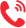更多 >>

学校：
地区：

北京大学
• 专业名称
• 所属学院
• 学制
• 学费(元)
• 地区
• 简章详情
• 报名
北京师范大学
• 专业名称
• 所属学院
• 学制
• 学费(元)
• 地区
• 简章详情
• 报名
大连理工大学
• 专业名称
• 所属学院
• 学制
• 学费(元)
• 地区
• 简章详情
• 报名
上海交通大学
• 专业名称
• 所属学院
• 学制
• 学费(元)
• 地区
• 简章详情
• 报名
武汉大学
• 专业名称
• 所属学院
• 学制
• 学费(元)
• 地区
• 简章详情
• 报名
兰州大学
• 专业名称
• 所属学院
• 学制
• 学费(元)
• 地区
• 简章详情
• 报名
北京交通大学
• 专业名称
• 所属学院
• 学制
• 学费(元)
• 地区
• 简章详情
• 报名
北京邮电大学
• 专业名称
• 所属学院
• 学制
• 学费(元)
• 地区
• 简章详情
• 报名
天津理工大学
• 专业名称
• 所属学院
• 学制
• 学费(元)
• 地区
• 简章详情
• 报名
河北大学
• 专业名称
• 所属学院
• 学制
• 学费(元)
• 地区
• 简章详情
• 报名
长春工业大学
• 专业名称
• 所属学院
• 学制
• 学费(元)
• 地区
• 简章详情
• 报名
常州大学
• 专业名称
• 所属学院
• 学制
• 学费(元)
• 地区
• 简章详情
• 报名
南昌航空大学
• 专业名称
• 所属学院
• 学制
• 学费(元)
• 地区
• 简章详情
• 报名
河南科技大学
• 专业名称
• 所属学院
• 学制
• 学费(元)
• 地区
• 简章详情
• 报名
湖北大学
• 专业名称
• 所属学院
• 学制
• 学费(元)
• 地区
• 简章详情
• 报名
湖南科技大学
• 专业名称
• 所属学院
• 学制
• 学费(元)
• 地区
• 简章详情
• 报名
长沙理工大学
• 专业名称
• 所属学院
• 学制
• 学费(元)
• 地区
• 简章详情
• 报名
中南林业科技大学
• 专业名称
• 所属学院
• 学制
• 学费(元)
• 地区
• 简章详情
• 报名
桂林电子科技大学
• 专业名称
• 所属学院
• 学制
• 学费(元)
• 地区
• 简章详情
• 报名
陕西科技大学
• 专业名称
• 所属学院
• 学制
• 学费(元)
• 地区
• 简章详情
• 报名
兰州理工大学
• 专业名称
• 所属学院
• 学制
• 学费(元)
• 地区
• 简章详情
• 报名
安徽建筑大学
• 专业名称
• 所属学院
• 学制
• 学费(元)
• 地区
• 简章详情
• 报名
扬州大学
• 专业名称
• 所属学院
• 学制
• 学费(元)
• 地区
• 简章详情
• 报名
北京电影学院
• 专业名称
• 所属学院
• 学制
• 学费(元)
• 地区
• 简章详情
• 报名
上海音乐学院
• 专业名称
• 所属学院
• 学制
• 学费(元)
• 地区
• 简章详情
• 报名

中国人民大学
• 专业名称
• 所属学院
• 学制
• 学费(元)
• 地区
• 简章详情
• 报名

更多 >>

更多 >>

## 联系方式400-101-7010

报名咨询热线

400-101-7010